## Business Case for Artificial Intelligence in the Plastic Injection Molding Business

Author: Chitta Ranjan, Ph.D., Director of Science, ProcessMiner, Inc.

Industry: Plastics

### OVERVIEW

Quality Control and High Scrap Rates for an F500 Plastic Injection Molding Manufacturer.

### BACKGROUND

A leading plastic injection molding factory was experiencing unusually high defect rates in a specialty plastic bottle designed for the healthcare industry. They were unable to identify or determine the root causes associated with these unusually high defect rates.

### RECOMMENDATION

ProcessMiner AutoPilot™ – In June 2020, during the height of the first wave of COVID-19 infections, a well-known manufacturer of plastic bottles agreed to pilot ProcessMiner’s turn-key AI platform for their plastic injection molding operations.

### IMPLEMENTATION

Positioned as a proof-of-concept, this pilot was conducted to determine whether Artificial Intelligence could help monitor, predict and prevent several common defects that were driving scrap rates upwards of 20 percent which is higher than the company average.

ProcessMiner quickly got to work studying data from more than 300 sensors on the plastic injection molding machine.

During the first 90 days of the pilot, [we] successfully gained a deep understanding of the different data parameters and the relationship they had on product quality for the bottle identified for the pilot period.

An agreement was reached to focus on two specific defect types that were driving 80 percent of the scrap for the specialty bottle being manufactured.

### SOLUTION

The project began with some offline data analysis that was shared with the customer to quickly identify the relative health of data quality for the pilot machine. After learning about the manufacturing process and the importance of dominant parameter control settings, the platform quickly delivered measurable value.

Through artificial intelligence, operators were given recommendations on ideal process control settings for dominant parameters, including temperature, pressure, and dryer settings. The AI-powered ideal parameter control recommendations and the pilot were successful in a 25 percent reduction in scrap rates.

#### At the end of the first phase of the pilot, the manufacturer had access to real-time monitoring, predictive analytics, and prescriptive parameter control recommendations that were automatically and securely sent to the machine control system.

Next, ProcessMiner deployed AutoPilot™ – an autonomous control feature of the platform that allows parameter control changes to be made automatically on the injection molding machine using IIoT technologies.

This closed-loop approach of machines operating machines delivered improved productivity, fewer defects, better productivity, and higher first-pass yield. This reduction in defects combined with the improvement in yield drove an annualized six-figure operational savings to the manufacturing operation.

Over the course of the pilot, the platform was also successful in autonomously driving the proper center-line parameters on the machine at the beginning of each new manufacturing shift.  This helped to drive down the variability that was being captured during the earlier phases of the pilot and helped the manufacturer operate in a more predictable environment.

## Artificial Intelligence Platform Achieves Unprecedented Autonomous Chemistry Control for Tissue Mill

Author: Chitta Ranjan, Ph.D., Director of Science, ProcessMiner, Inc.

Industry: Chemicals, Pulp & Paper

## Overview

The goal of this project was to autonomously control part of a tissue mill’s continuous manufacturing process using artificial intelligence and predictive analytics to reduce raw material consumption while maintaining the product quality with specification limit.

Predictive analytics uses a manufacturing machine’s historical data to predict process outcomes. Through machine learning and artificial intelligence, these models continue to learn, adapt and evolve, providing the user with accurate information that can drive better decisions.

## Challenge

It is difficult to learn a multitude of relationships in a complex manufacturing environment. Typically, an operator uses his or her past experiences in determining how to regulate the dosage of a raw material with:

• preferred minimum raw material consumed, while
• keeping the product quality within range

The challenge, therein, lies with the operator’s ability to act quickly with the dynamically changing manufacturing processes, and deliver continuous process improvement with autonomous chemistry control.

## Solution

This is where the ProcessMiner AutoPilot real-time predictive system comes in and solves the problem, making recommendations and prescribing solutions. In doing this, it minimizes raw materials and reduces costs while maintaining both speed and product quality.

ProcessMiner’s AI platform is a cloud-based system that utilizes information, including:

• Physical tissue/papermaker property measurements
• Tissue/papermaking process knowledge
• System ‘meta-data’ to mathematically model the tissue/papermaker system

The information generated by this continuously-learning model is then used to accurately predict key performance indicators (KPI) of the tissue/paper machine, and therefore, provide actionable insights.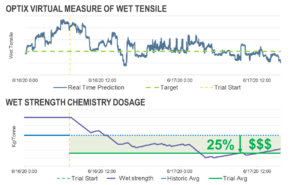The Solenis OPTIX ProcessMiner AutoPilot platform is designed to be an adaptive and evolving artificial intelligence system, allowing it to automatically and simultaneously learn these relationships. The adaptive analytics system accurately learns complex variable relationships in pulp and paper manufacturing processes and yields a digital measure of product quality.

For example: every time the system hits a new data point, it runs an orchestration (sequence of steps) series of processes, and in turn, updates the AI platform to adapt and evolve, always morphing to holistically make recommendations in real-time.

### During the onboarding process, the ProcessMiner system loads up to one year of historical data, uses that past data to immediately initialize and train its artificial system so it is ready to go as soon as it is launched.

About the ProcessMiner AutoPilot adaptive and evolving model: Tissue/Paper manufacturing is a complex, continuous process that slowly and sometimes abruptly changes over time.

Example: When conditions in the complex tissue/paper manufacturing processes change over time due to parameter control variations (e.g. changes in raw materials or machine speed) it’s difficult to predict the quality of the produced product ahead of lab tests and often leads to machine operators making reactive control adjustments.

• These scenarios lead to two considerations, namely the predictive model needs to be updated frequently and historical data quickly becomes obsolete
• Thus, a continuously-evolving and adaptive model is required
• The ProcessMiner AutoPilot predictive model evolves over time as more data is collected
• With new data streaming, the old data slowly loses its relevance
• The prediction system is built such that it automatically uses the knowledge gathered from the old data and combines it with the new data to construct an evolved (a relearned and more accurate) model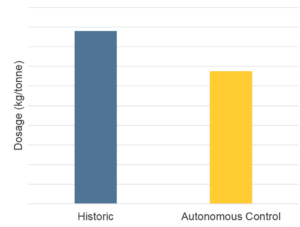Additionally, the prediction system self-adapts to a process change.  For the model adaptation, we perform the best prediction model selection, and its retuning, periodically and on-demand.

• The periodic runs are to ensure nothing is missed by the triggers
• The on-demand is triggered automatically whenever a process shift is detected
• Data with measurement errors are identified in real-time using F-tests and are isolated from the training data
• Both of the above features are computationally intensive
• To deploy them in real-time, we parallelized the processes through multiple processing units
• The parallel processing enables the delivery of accurate predictions in real-time every 30 seconds.

The charts throughout this case study show the outcome of a predictive model that uses an off-the-shelf approach without considering the unique characteristics of the tissue/papermaking process compared with a model using a tailor-made, advanced approach such as the one discussed previously.  The oversimplified model depicted on the left does not mirror the true process, while that on the right considers the complexity of the tissue/papermaking process and clearly delivers predictions that are more accurate.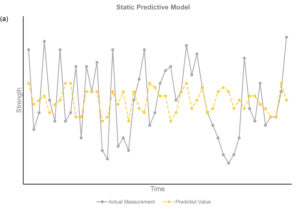• A predictive model using poor prediction techniques that oversimplify the process (left)
• A predictive model with the same data utilizing advanced techniques capable of handling paper machine process data (right)

Predictive Model Accuracy: An accurate model provides predictions close to the actual value most of the time. The absolute value of the prediction, minus the test value, should be close to zero. Prediction accuracy must also be measured in real-time to instill confidence in users acting on the predictions. One method to evaluate prediction accuracy in real-time is to use a control chart to monitor the difference between actual and predicted values.

What makes the platform unique?

• Predictive soft sensor
• Machine learning
• Application expertise
• Model diagnostics
• Insight to influential process variables

Results

The results are a manufacturing game-changer. Autonomous manufacturing using AI with machine learning allows for improved product quality, optimized use of raw materials with reduced water and energy consumption.

Using a closed-loop controller in conjunction with quality parameter predictions, the mill was able to control its strength chemistry autonomously to ensure optimal chemical feed and adhere to target parameters.

As a result of the artificial intelligence platform, the tissue mill achieved unprecedented autonomous chemistry, allowing for:

• Besides Wet Strength, Also Reduced Dry Strength Chemistry by 25%
• Grade ~30% Wet Strength Chemistry## LSTM Autoencoder for Extreme Rare Event Classification in Keras

By: Chitta Ranjan, Ph.D., Director of Science, ProcessMiner, Inc.

Here we will learn the details of data preparation for LSTM models, and build an LSTM Autoencoder for rare-event classification.

This post is a continuation of my previous post Extreme Rare Event Classification using Autoencoders. In the previous post, we talked about the challenges in an extremely rare event data with less than 1% positively labeled data. We built an Autoencoder Classifier for such processes using the concepts of Anomaly Detection.

However, the data we have is a time series. But earlier we used a Dense layer Autoencoder that does not use the temporal features in the data. Therefore, in this post, we will improve on our approach by building an LSTM Autoencoder.

Here, we will learn:

• data preparation steps for an LSTM model,
• building and implementing LSTM autoencoder, and
• using LSTM autoencoder for rare-event classification.

Quick recap on LSTM:

• LSTM is a type of Recurrent Neural Network (RNN). RNNs, in general, and LSTM, specifically, are used on sequential or time series data.
• These models are capable of automatically extracting effect of past events.
• LSTM are known for its ability to extract both long- and short- term effects of pasts event.

In the following, we will go directly to developing an LSTM Autoencoder. It is recommended to read Step-by-step understanding LSTM Autoencoder layers to better understand and further improve the network below.

About the data problem in brief, we have real-world data on sheet breaks from a paper manufacturing. Our objective is to predict the break in advance. Please refer to Extreme Rare Event Classification using Autoencoders for the details on the data, problem, and the classification approach.

# LSTM Autoencoder for Multivariate Data

In our problem, we have a multivariate time-series data. A multivariate time-series data contains multiple variables observed over a period of time. We will build an LSTM autoencoder on this multivariate time-series to perform rare-event classification. As described in , this is achieved by using an anomaly detection approach:

• we build an autoencoder on the normal (negatively labeled) data,
• use it to reconstruct a new sample,
• if the reconstruction error is high, we label it as a sheet-break.

LSTM requires few special data-preprocessing steps. In the following, we will give sufficient attention to these steps.

Let’s get to the implementation.

# Libraries

I like to put together the libraries and global constants first.

```%matplotlib inline
import matplotlib.pyplot as plt
import seaborn as sns

import pandas as pd
import numpy as np
from pylab import rcParams

import tensorflow as tf
from keras import optimizers, Sequential
from keras.models import Model
from keras.utils import plot_model
from keras.layers import Dense, LSTM, RepeatVector, TimeDistributed
from keras.callbacks import ModelCheckpoint, TensorBoard

from sklearn.preprocessing import StandardScaler
from sklearn.model_selection import train_test_split
from sklearn.metrics import confusion_matrix, precision_recall_curve
from sklearn.metrics import recall_score, classification_report, auc, roc_curve
from sklearn.metrics import precision_recall_fscore_support, f1_score

from numpy.random import seed
seed(7)
from tensorflow import set_random_seed
set_random_seed(11)from sklearn.model_selection import train_test_split

SEED = 123 #used to help randomly select the data points
DATA_SPLIT_PCT = 0.2

rcParams['figure.figsize'] = 8, 6
LABELS = ["Normal","Break"]```

# Data Preparation

As mentioned before, LSTM requires a few specific steps in the data preparation. The input to LSTMs are 3-dimensional arrays created from the time-series data. This is an error prone step so we will look at the details.

The data is taken from . The link to the data is here.

```df = pd.read_csv("data/processminer-rare-event-mts - data.csv")

## Curve Shifting

As also mentioned in , the objective of this rare-event problem is to predict a sheet-break before it occurs. We will try to predict the break up to 4 minutes in advance. For this data, this is equivalent to shifting the labels up by two rows. It can be done directly with `df.y=df.y.shift(-2)`. However, here we require to do the following,

• For any row n with label 1, make (n-2):(n-1) as 1. With this, we are teaching the classifier to predict up to 4 minutes ahead. And,
• remove row n. Row n is removed because we are not interested in teaching the classifier to predict a break when it has already happened.

We develop the following function to perform this curve shifting.

```sign = lambda x: (1, -1)[x < 0]

def curve_shift(df, shift_by):
'''
This function will shift the binary labels in a dataframe.
The curve shift will be with respect to the 1s.
For example, if shift is -2, the following process
will happen: if row n is labeled as 1, then
- Make row (n+shift_by):(n+shift_by-1) = 1.
- Remove row n.
i.e. the labels will be shifted up to 2 rows up.

Inputs:
df       A pandas dataframe with a binary labeled column.
This labeled column should be named as 'y'.
shift_by An integer denoting the number of rows to shift.

Output
df       A dataframe with the binary labels shifted by shift.
'''

vector = df['y'].copy()
for s in range(abs(shift_by)):
tmp = vector.shift(sign(shift_by))
tmp = tmp.fillna(0)
vector += tmp
labelcol = 'y'
# Add vector to the df
df.insert(loc=0, column=labelcol+'tmp', value=vector)
# Remove the rows with labelcol == 1.
df = df.drop(df[df[labelcol] == 1].index)
# Drop labelcol and rename the tmp col as labelcol
df = df.drop(labelcol, axis=1)
df = df.rename(columns={labelcol+'tmp': labelcol})
# Make the labelcol binary
df.loc[df[labelcol] > 0, labelcol] = 1

return df```

We will now shift our data and verify if the shifting is correct. In the subsequent sections, we have few more test steps. It is recommended to use them to ensure the data preparation steps are working as expected.

```print('Before shifting')  # Positive labeled rows before shifting.
one_indexes = df.index[df['y'] == 1]
display(df.iloc[(np.where(np.array(input_y) == 1)-5):(np.where(np.array(input_y) == 1)+1), ])

# Shift the response column y by 2 rows to do a 4-min ahead prediction.
df = curve_shift(df, shift_by = -2)

print('After shifting')  # Validating if the shift happened correctly.

If we note here, we moved the positive label at 5/1/99 8:38 to n-1 and n-2 timestamps, and dropped row n. Also, there is a time difference of more than 2 minutes between a break row and the next row. This is because, when a break occurs, the machine stays in the break status for a while. During this time, we have y = 1 for consecutive rows. In the provided data, these consecutive break rows are deleted to prevent the classifier from learning to predict a break after it has already happened. Refer  for details.

Before moving forward, we clean up the data by dropping the time, and two other categorical columns.

```# Remove time column, and the categorical columns
df = df.drop(['time', 'x28', 'x61'], axis=1)```

## Prepare Input Data for LSTM

LSTM is a bit more demanding than other models. Significant amount of time and attention may go in preparing the data that fits an LSTM. However, it is generally worth the effort.

The input data to an LSTM model is a 3-dimensional array. The shape of the array is samples x lookback x features. Let’s understand them,

• samples: This is simply the number of observations, or in other words, the number of data points.
• lookback: LSTM models are meant to look at the past. Meaning, at time the LSTM will process data up to (tlookback) to make a prediction.
• features: It is the number of features present in the input data.

First, we will extract the features and response.

```input_X = df.loc[:, df.columns != 'y'].values  # converts the df to a numpy array
input_y = df['y'].values

n_features = input_X.shape  # number of features```

The `input_X` here is a 2-dimensional array of size samples x features. We want to be able to transform such a 2D array into a 3D array of size: samples x lookback x features. Refer to Figure 1 above for a visual understanding.

For that, we develop a function `temporalize` .

```def temporalize(X, y, lookback):
'''
Inputs
X         A 2D numpy array ordered by time of shape:
(n_observations x n_features)
y         A 1D numpy array with indexes aligned with
X, i.e. y[i] should correspond to X[i].
Shape: n_observations.
lookback  The window size to look back in the past
records. Shape: a scalar.

Output
output_X  A 3D numpy array of shape:
((n_observations-lookback-1) x lookback x
n_features)
output_y  A 1D array of shape:
(n_observations-lookback-1), aligned with X.
'''
output_X = []
output_y = []
for i in range(len(X) - lookback - 1):
t = []
for j in range(1, lookback + 1):
# Gather the past records upto the lookback period
t.append(X[[(i + j + 1)], :])
output_X.append(t)
output_y.append(y[i + lookback + 1])
return np.squeeze(np.array(output_X)), np.array(output_y)```

To test and demonstrate this function, we will look at an example below with `lookback = 5` .

```print('First instance of y = 1 in the original data')
display(df.iloc[(np.where(np.array(input_y) == 1)-5):(np.where(np.array(input_y) == 1)+1), ])lookback = 5  # Equivalent to 10 min of past data.
# Temporalize the data
X, y = temporalize(X = input_X, y = input_y, lookback = lookback)print('For the same instance of y = 1, we are keeping past 5 samples in the 3D predictor array, X.')
display(pd.DataFrame(np.concatenate(X[np.where(np.array(y) == 1)], axis=0 )))```

What we are looking for here is,

• In the original data, y = 1 at row 257.
• With `lookback = 5` we want the LSTM to look at the 5 rows before row 257 (including itself).
• In the 3D array, `X`, each 2D block at `X[i,:,:]` denotes the prediction data that corresponds to `y[i]` . To draw an analogy, in regression `y[i]` corresponds to a 1D vector `X[i,:]` ; in LSTM `y[i]` corresponds to a 2D array `X[i,:,:]` .
• This 2D block `X[i,:,:]` should have the predictors at `input_X[i,:]` and the previous rows up to the given `lookback` .
• As we can see in the output above, the `X[i,:,:]` block in the bottom is the same as the five past rows of y=1 shown on the top.
• Similarly, this is applied for the entire data, for all y’s. The example here is shown for an instance of y=1 for easier visualization.

# Split into train, valid, and test

This is straightforward with the `sklearn` function.

`X_train, X_test, y_train, y_test = train_test_split(np.array(X), np.array(y), test_size=DATA_SPLIT_PCT, random_state=SEED)X_train, X_valid, y_train, y_valid = train_test_split(X_train, y_train, test_size=DATA_SPLIT_PCT, random_state=SEED)`

For training the autoencoder, we will be using the X coming from only the negatively labeled data. Therefore, we separate the X corresponding to y = 0.

```X_train_y0 = X_train[y_train==0]
X_train_y1 = X_train[y_train==1]X_valid_y0 = X_valid[y_valid==0]
X_valid_y1 = X_valid[y_valid==1]```

We will reshape the X’s into the required 3D dimension: sample x lookback x features.

```X_train = X_train.reshape(X_train.shape, lookback, n_features)
X_train_y0 = X_train_y0.reshape(X_train_y0.shape, lookback, n_features)
X_train_y1 = X_train_y1.reshape(X_train_y1.shape, lookback, n_features)X_valid = X_valid.reshape(X_valid.shape, lookback, n_features)
X_valid_y0 = X_valid_y0.reshape(X_valid_y0.shape, lookback, n_features)
X_valid_y1 = X_valid_y1.reshape(X_valid_y1.shape, lookback, n_features)X_test = X_test.reshape(X_test.shape, lookback, n_features)```

## Standardize the Data

It is usually better to use a standardized data (transformed to Gaussian with mean 0 and standard deviation 1) for autoencoders.

One common standardization mistake is: we normalize the entire data and then split into train-test. This is incorrect. Test data should be completely unseen to anything during the modeling. We should, therefore, normalize the training data, and use its summary statistics to normalize the test data (for normalization, these statistics are the mean and variances of each feature).

Standardizing this data is a bit tricky. This is because the X matrices are 3D, and we want the standardization to happen with respect to the original 2D data.

To do this, we will require two UDFs.

• `flatten` : This function will re-create the original 2D array from which the 3D arrays were created. This function is the inverse of `temporalize`, meaning `X = flatten(temporalize(X))`.
• `scale` : This function will scale a 3D array that we created as inputs to the LSTM.
```def flatten(X):
'''
Flatten a 3D array.

Input
X            A 3D array for lstm, where the array is sample x timesteps x features.

Output
flattened_X  A 2D array, sample x features.
'''
flattened_X = np.empty((X.shape, X.shape))  # sample x features array.
for i in range(X.shape):
flattened_X[i] = X[i, (X.shape-1), :]
return(flattened_X)

def scale(X, scaler):
'''
Scale 3D array.

Inputs
X            A 3D array for lstm, where the array is sample x timesteps x features.
scaler       A scaler object, e.g., sklearn.preprocessing.StandardScaler, sklearn.preprocessing.normalize

Output
X            Scaled 3D array.
'''
for i in range(X.shape):
X[i, :, :] = scaler.transform(X[i, :, :])

return X```

Why didn’t we first normalize the original 2D data and then create the 3D arrays? Because, to do this we will: split the data into train and test, followed by their normalization. However, when we create the 3D arrays on the test data, we lose the initial rows of samples up till the lookback. Splitting into train-valid-test will cause this for both the validation and test sets.

We will fit a Standardization object from `sklearn`. This function standardizes the data to Normal(0, 1). Note that we require to flatten the `X_train_y0` array to pass to the `fit` function.

```# Initialize a scaler using the training data.
scaler = StandardScaler().fit(flatten(X_train_y0))```

We will use our UDF, `scale`, to standardize `X_train_y0` with the fitted transform object `scaler`.

`X_train_y0_scaled = scale(X_train_y0, scaler)`

Make sure the `scale` worked correctly?

A correct transformation of `X_train` will ensure that the means and variances of each column of the flattened `X_train` are 0 and 1, respectively. We test this.

```a = flatten(X_train_y0_scaled)
print('colwise mean', np.mean(a, axis=0).round(6))
print('colwise variance', np.var(a, axis=0))```

All the means and variances outputted above are 0 and 1, respectively. Therefore, the scaling is correct. We will now scale the validation and test sets. We will again use the `scaler` object on these sets.

```X_valid_scaled = scale(X_valid, scaler)
X_valid_y0_scaled = scale(X_valid_y0, scaler)X_test_scaled = scale(X_test, scaler)```

# LSTM Autoencoder Training

We will, first, initialize few variables.

```timesteps =  X_train_y0_scaled.shape # equal to the lookback
n_features =  X_train_y0_scaled.shape # 59

epochs = 200
batch = 64
lr = 0.0001```

We, now, develop a simple architecture.

```lstm_autoencoder = Sequential()
# Encoder
# Decoder

lstm_autoencoder.summary()```

From the summary(), the total number of parameters are 5,331. This is about half of the training size. Hence, this is an appropriate model to fit. To have a bigger architecture, we will need to add regularization, e.g. Dropout, which will be covered in the next post.

Now, we will train the autoencoder.

```adam = optimizers.Adam(lr)

cp = ModelCheckpoint(filepath="lstm_autoencoder_classifier.h5",
save_best_only=True,
verbose=0)

tb = TensorBoard(log_dir='./logs',
histogram_freq=0,
write_graph=True,
write_images=True)

lstm_autoencoder_history = lstm_autoencoder.fit(X_train_y0_scaled, X_train_y0_scaled,
epochs=epochs,
batch_size=batch,
validation_data=(X_valid_y0_scaled, X_valid_y0_scaled),
verbose=2).history```

Plotting the change in the loss over the epochs.

```plt.plot(lstm_autoencoder_history['loss'], linewidth=2, label='Train')
plt.plot(lstm_autoencoder_history['val_loss'], linewidth=2, label='Valid')
plt.legend(loc='upper right')
plt.title('Model loss')
plt.ylabel('Loss')
plt.xlabel('Epoch')
plt.show()```

## Classification

Similar to the previous post , here we show how we can use an Autoencoder reconstruction error for the rare-event classification. We follow this concept: the autoencoder is expected to reconstruct a noif the reconstruction error is high, we will classify it as a sheet-break.

We will need to determine the threshold for this. Also, note that here we will be using the entire validation set containing both y = 0 or 1.

```valid_x_predictions = lstm_autoencoder.predict(X_valid_scaled)
mse = np.mean(np.power(flatten(X_valid_scaled) - flatten(valid_x_predictions), 2), axis=1)

error_df = pd.DataFrame({'Reconstruction_error': mse,
'True_class': y_valid.tolist()})

precision_rt, recall_rt, threshold_rt = precision_recall_curve(error_df.True_class, error_df.Reconstruction_error)
plt.plot(threshold_rt, precision_rt[1:], label="Precision",linewidth=5)
plt.plot(threshold_rt, recall_rt[1:], label="Recall",linewidth=5)
plt.title('Precision and recall for different threshold values')
plt.xlabel('Threshold')
plt.ylabel('Precision/Recall')
plt.legend()
plt.show()```

Note that we have to `flatten` the arrays to compute the `mse`.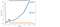Figure 3. A threshold of 0.3 should provide a reasonable trade-off between precision and recall, as we want to higher recall.

Now, we will perform classification on the test data.

We should not estimate the classification threshold from the test data. It will result in overfitting.

```test_x_predictions = lstm_autoencoder.predict(X_test_scaled)
mse = np.mean(np.power(flatten(X_test_scaled) - flatten(test_x_predictions), 2), axis=1)

error_df = pd.DataFrame({'Reconstruction_error': mse,
'True_class': y_test.tolist()})

threshold_fixed = 0.3
groups = error_df.groupby('True_class')
fig, ax = plt.subplots()

for name, group in groups:
ax.plot(group.index, group.Reconstruction_error, marker='o', ms=3.5, linestyle='',
label= "Break" if name == 1 else "Normal")
ax.hlines(threshold_fixed, ax.get_xlim(), ax.get_xlim(), colors="r", zorder=100, label='Threshold')
ax.legend()
plt.title("Reconstruction error for different classes")
plt.ylabel("Reconstruction error")
plt.xlabel("Data point index")
plt.show();```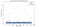Figure 4. Using threshold = 0.8 for classification. The orange and blue dots above the threshold line represents the True Positive and False Positive, respectively.

In Figure 4, the orange and blue dot above the threshold line represents the True Positive and False Positive, respectively. As we can see, we have good number of false positives.

Let’s see the accuracy results.

## Test Accuracy

Confusion Matrix

```pred_y = [1 if e > threshold_fixed else 0 for e in error_df.Reconstruction_error.values]conf_matrix = confusion_matrix(error_df.True_class, pred_y)

plt.figure(figsize=(6, 6))
sns.heatmap(conf_matrix, xticklabels=LABELS, yticklabels=LABELS, annot=True, fmt="d");
plt.title("Confusion matrix")
plt.ylabel('True class')
plt.xlabel('Predicted class')
plt.show()```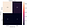Figure 5. Confusion matrix showing the True Positives and False Positives.

ROC Curve and AUC

```false_pos_rate, true_pos_rate, thresholds = roc_curve(error_df.True_class, error_df.Reconstruction_error)
roc_auc = auc(false_pos_rate, true_pos_rate,)

plt.plot(false_pos_rate, true_pos_rate, linewidth=5, label='AUC = %0.3f'% roc_auc)
plt.plot([0,1],[0,1], linewidth=5)

plt.xlim([-0.01, 1])
plt.ylim([0, 1.01])
plt.legend(loc='lower right')
plt.ylabel('True Positive Rate')
plt.xlabel('False Positive Rate')
plt.show()```

We see approximately 10% improvement in the AUC compared to the dense layer Autoencoder in . From the Confusion Matrix in Figure 5, we could predict 10 out of 39 break instances. As also discussed in , this is significant for a paper mill. However, the improvement we achieved in comparison to the dense layer Autoencoder is minor.

The primary reason is LSTM model has more parameters to estimate. It becomes important to use regularization with LSTMs. Regularization and other model improvements will be discussed in the next post.

# What can be done better?

In the next article, we will learn tuning an Autoencoder. We will go over,

• CNN LSTM Autoencoder,
• Dropout layer,
• LSTM Dropout (Dropout_U and Dropout_W)
• Gaussian-dropout layer
• SELU activation, and
• alpha-dropout with SELU activation.

# Conclusion

This post continued the work on extreme rare event binary labeled data in . To utilize the temporal patterns, LSTM Autoencoders is used to build a rare event classifier for a multivariate time-series process. Details about the data preprocessing steps for LSTM model are discussed. A simple LSTM Autoencoder model is trained and used for classification. Some improvement in the accuracy over a Dense Autoencoder is found. For further improvement, we will look at ways to improve an Autoencoder with Dropout and other techniques in the next post.

## Extreme Rare Event Classification using Autoencoders in Keras

By: Chitta RanjanPh.D., Director of Science, ProcessMiner, Inc.

### Background: What is an extreme rare event?

In a rare-event problem, we have an unbalanced dataset. Meaning, we have fewer positively labeled samples than negative. In a typical rare-event problem, the positively labeled data are around 5–10% of the total. In an extremely rare event problem, we have less than 1% positively labeled data. For example, in the dataset used here, it is around 0.6%.

Such extreme rare event problems are quite common in the real-world, for example, sheet-breaks and machine failure in manufacturing, clicks, or purchase in the online industry.

Classifying these rare events is quite challenging. Recently, Deep Learning has been quite extensively used for classification. However, the small number of positively labeled samples prohibits Deep Learning applications. No matter how large the data, the use of Deep Learning gets limited by the amount of positively labeled samples.

### Why should we still bother to use Deep Learning?

This is a legitimate question. Why should we not think of using some another Machine Learning approach?

The answer is subjective. We can always go with a Machine Learning approach. To make it work, we can undersample from negatively labeled data to have a close to a balanced dataset. Since we have about 0.6% positively labeled data, the undersampling will result in rougly a dataset that is about 1% of the size of the original data. A Machine Learning approach, e.g. SVM or Random Forest, will still work on a dataset of this size. However, it will have limitations in its accuracy. And we will not utilize the information present in the remaining ~99% of the data.

If the data is sufficient, Deep Learning methods are potentially more capable. It also allows flexibility for model improvement by using different architectures. We will, therefore, attempt to use Deep Learning methods.

In this post, we will learn how we can use a simple dense layers autoencoder to build a rare event classifier. The purpose of this post is to demonstrate the implementation of an Autoencoder for extreme rare-event classification. We will leave the exploration of different architecture and configuration of the Autoencoder on the user. Please share in the comments if you find anything interesting.

### Autoencoder for Classification

The autoencoder approach for classification is similar to anomaly detection. In anomaly detection, we learn the pattern of a normal process. Anything that does not follow this pattern is classified as an anomaly. For a binary classification of rare events, we can use a similar approach using autoencoders (derived from here ).

#### Quick revision: What is an autoencoder?

• An autoencoder is made of two modules: encoder and decoder
• The encoder learns the underlying features of a process; these features are typically in a reduced dimension
• The decoder can recreate the original data from these underlying features

### How to use an Autoencoder rare-event classification?

• We will divide the data into two parts: positively labeled and negatively labeled
• The negatively labeled data is treated as a normal state of the process; A normal state is when the process is relentless
• We will ignore the positively labeled data, and train an Autoencoder on only negatively labeled data
• This Autoencoder has now learned the features of the normal process
• A well-trained Autoencoder will predict any new data that is coming from the normal state of the process (as it will have the same pattern or distribution)
• Therefore, the reconstruction error will be small
• However, if we try to reconstruct data from a rare-event, the Autoencoder will struggle
• This will make the reconstruction error high during the rare-event
• We can catch such high reconstruction errors and label them as a rare-event prediction
• This procedure is similar to anomaly detection methods

### Implementation: Data and Problem

This is a binary labeled data from a pulp-and-paper mill for sheet breaks. Sheet breaks is a severe problem in paper manufacturing. A single sheet break causes a loss of several thousand dollars, and the mills see at least one or more breaks every day. This causes millions of dollars of yearly losses and work hazards.

Detecting a break event is challenging due to the nature of the process. As mentioned in , even a 5% reduction in the breaks will bring significant benefit to the mills.

The data we have contains about 18k rows collected over 15 days. The column contains the binary labels, with 1 denoting a sheet break. The rest columns are predictors. There are about 124 positive labeled samples (~0.6%).

### Code: Import the desired libraries.

```%matplotlib inline
import matplotlib.pyplot as plt
import seaborn as snsimport pandas as pd
import numpy as np
from pylab import rcParamsimport tensorflow as tf
from keras.layers import Input, Dense
from keras.callbacks import ModelCheckpoint, TensorBoard
from keras import regularizersfrom sklearn.preprocessing import StandardScaler
from sklearn.model_selection import train_test_split
from sklearn.metrics import confusion_matrix, precision_recall_curve
from sklearn.metrics import recall_score, classification_report, auc, roc_curve
from sklearn.metrics import precision_recall_fscore_support, f1_scorefrom numpy.random import seed
seed(1)
from tensorflow import set_random_seed
set_random_seed(2)SEED = 123 #used to help randomly select the data points
DATA_SPLIT_PCT = 0.2rcParams['figure.figsize'] = 8, 6
LABELS = ["Normal","Break"]```

Note that we are setting the random seeds for the reproducibility of the result.

##### Data Preprocessing

Now, we read and prepare the data.[/vc_column_text][vc_column_text]

`df = pd.read_csv("data/processminer-rare-event-mts - data.csv")`

The objective of this rare-event problem is to predict a sheet-break before it occurs. We will try to predict the break 4 minutes in advance. To build this model, we will shift the labels 2 rows up (which corresponds to 4 minutes). We can do this as:  However, in this problem, we would want to do the shifting as: if row n is positively labeled:

• make rows (n-2) and (n-1) equal to 1 | This will help the classifier learn up to 4 minutes ahead prediction
• Delete row n, bsecause we do not want the classifier to learn to predict a break when it has happened

We will develop the following UDF for this curve shifting.[/vc_column_text][vc_column_text]

```sign = lambda x: (1, -1)[x < 0]

def curve_shift(df, shift_by):
'''
This function will shift the binary labels in a dataframe.
The curve shift will be with respect to the 1s.
For example, if shift is -2, the following process
will happen: if row n is labeled as 1, then
- Make row (n+shift_by):(n+shift_by-1) = 1.
- Remove row n.
i.e. the labels will be shifted up to 2 rows up.

Inputs:
df       A pandas dataframe with a binary labeled column.
This labeled column should be named as 'y'.
shift_by An integer denoting the number of rows to shift.

Output
df       A dataframe with the binary labels shifted by shift.
'''

vector = df['y'].copy()
for s in range(abs(shift_by)):
tmp = vector.shift(sign(shift_by))
tmp = tmp.fillna(0)
vector += tmp
labelcol = 'y'
# Add vector to the df
df.insert(loc=0, column=labelcol+'tmp', value=vector)
# Remove the rows with labelcol == 1.
df = df.drop(df[df[labelcol] == 1].index)
# Drop labelcol and rename the tmp col as labelcol
df = df.drop(labelcol, axis=1)
df = df.rename(columns={labelcol+'tmp': labelcol})
# Make the labelcol binary
df.loc[df[labelcol] > 0, labelcol] = 1

return df```

Before moving forward, we will drop the time, and also the categorical columns for simplicity.

```# Remove time column, and the categorical columns
df = df.drop(['time', 'x28', 'x61'], axis=1)```

Now, we divide the data into train, valid, and test sets. Then we will take the subset of data with only 0s to train the autoencoder.

```df_train, df_test = train_test_split(df, test_size=DATA_SPLIT_PCT, random_state=SEED)
df_train, df_valid = train_test_split(df_train, test_size=DATA_SPLIT_PCT, random_state=SEED)df_train_0 = df_train.loc[df['y'] == 0]
df_train_1 = df_train.loc[df['y'] == 1]
df_train_0_x = df_train_0.drop(['y'], axis=1)
df_train_1_x = df_train_1.drop(['y'], axis=1)df_valid_0 = df_valid.loc[df['y'] == 0]
df_valid_1 = df_valid.loc[df['y'] == 1]
df_valid_0_x = df_valid_0.drop(['y'], axis=1)
df_valid_1_x = df_valid_1.drop(['y'], axis=1)df_test_0 = df_test.loc[df['y'] == 0]
df_test_1 = df_test.loc[df['y'] == 1]
df_test_0_x = df_test_0.drop(['y'], axis=1)
df_test_1_x = df_test_1.drop(['y'], axis=1)```

Standardization: It is usually better to use standardized data (transformed to Gaussian, mean 0 and variance 1) for autoencoders.

```scaler = StandardScaler().fit(df_train_0_x)
df_train_0_x_rescaled = scaler.transform(df_train_0_x)
df_valid_0_x_rescaled = scaler.transform(df_valid_0_x)
df_valid_x_rescaled = scaler.transform(df_valid.drop(['y'], axis = 1))df_test_0_x_rescaled = scaler.transform(df_test_0_x)
df_test_x_rescaled = scaler.transform(df_test.drop(['y'], axis = 1))```

### Autoencoder Classifier

```nb_epoch = 200
batch_size = 128
input_dim = df_train_0_x_rescaled.shape #num of predictor variables,
encoding_dim = 32
hidden_dim = int(encoding_dim / 2)
learning_rate = 1e-3

input_layer = Input(shape=(input_dim, ))
encoder = Dense(encoding_dim, activation="relu", activity_regularizer=regularizers.l1(learning_rate))(input_layer)
encoder = Dense(hidden_dim, activation="relu")(encoder)
decoder = Dense(hidden_dim, activation="relu")(encoder)
decoder = Dense(encoding_dim, activation="relu")(decoder)
decoder = Dense(input_dim, activation="linear")(decoder)
autoencoder = Model(inputs=input_layer, outputs=decoder)
autoencoder.summary()```

Training: We will train the model and save it in a file. Saving a trained model is a good practice for saving time for future analysis.

```autoencoder.compile(metrics=['accuracy'],
loss='mean_squared_error',
save_best_only=True,
verbose=0)tb = TensorBoard(log_dir='./logs',
histogram_freq=0,
write_graph=True,
write_images=True)history = autoencoder.fit(df_train_0_x_rescaled, df_train_0_x_rescaled,
epochs=nb_epoch,
batch_size=batch_size,
shuffle=True,
validation_data=(df_valid_0_x_rescaled, df_valid_0_x_rescaled),
verbose=1,
callbacks=[cp, tb]).history```

Classification: In the following, we show how we can use an Autoencoder reconstruction error for the rare-event classification.

As mentioned before, if the reconstruction error is high, we will classify it as a sheet-break. We will need to determine the threshold for this.

We will use the validation set to identify the threshold.

```valid_x_predictions = autoencoder.predict(df_valid_x_rescaled)
mse = np.mean(np.power(df_valid_x_rescaled - valid_x_predictions, 2), axis=1)
error_df = pd.DataFrame({'Reconstruction_error': mse,
'True_class': df_valid['y']})precision_rt, recall_rt, threshold_rt = precision_recall_curve(error_df.True_class, error_df.Reconstruction_error)
plt.plot(threshold_rt, precision_rt[1:], label="Precision",linewidth=5)
plt.plot(threshold_rt, recall_rt[1:], label="Recall",linewidth=5)
plt.title('Precision and recall for different threshold values')
plt.xlabel('Threshold')
plt.ylabel('Precision/Recall')
plt.legend()
plt.show()```

We should not estimate the classification threshold from the test data. It will result in overfitting.

```test_x_predictions = autoencoder.predict(df_test_x_rescaled)
mse = np.mean(np.power(df_test_x_rescaled - test_x_predictions, 2), axis=1)
error_df_test = pd.DataFrame({'Reconstruction_error': mse,
'True_class': df_test['y']})
error_df_test = error_df_test.reset_index()threshold_fixed = 0.4
groups = error_df_test.groupby('True_class')fig, ax = plt.subplots()for name, group in groups:
ax.plot(group.index, group.Reconstruction_error, marker='o', ms=3.5, linestyle='',
label= "Break" if name == 1 else "Normal")
ax.hlines(threshold_fixed, ax.get_xlim(), ax.get_xlim(), colors="r", zorder=100, label='Threshold')
ax.legend()
plt.title("Reconstruction error for different classes")
plt.ylabel("Reconstruction error")
plt.xlabel("Data point index")
plt.show();```
```pred_y = [1 if e > threshold_fixed else 0 for e in error_df.Reconstruction_error.values]conf_matrix = confusion_matrix(error_df.True_class, pred_y)plt.figure(figsize=(12, 12))
sns.heatmap(conf_matrix, xticklabels=LABELS, yticklabels=LABELS, annot=True, fmt="d");
plt.title("Confusion matrix")
plt.ylabel('True class')
plt.xlabel('Predicted class')
plt.show()```

We could predict 8 out of 41 breaks instances. Note that these instances include 2 or 4 minute ahead predictions. This is around 20%, which is a good recall rate for the paper industry. The False Positive Rate is around 6%. This is not ideal but not terrible for a mill.

Still, this model can be further improved to increase the recall rate with smaller False Positive Rate. We will look at the AUC below and then talk about the next approach for improvement.

##### ROC Curve and AUC
```false_pos_rate, true_pos_rate, thresholds = roc_curve(error_df.True_class, error_df.Reconstruction_error)
roc_auc = auc(false_pos_rate, true_pos_rate,)plt.plot(false_pos_rate, true_pos_rate, linewidth=5, label='AUC = %0.3f'% roc_auc)
plt.plot([0,1],[0,1], linewidth=5)plt.xlim([-0.01, 1])
plt.ylim([0, 1.01])
plt.legend(loc='lower right')
plt.ylabel('True Positive Rate')
plt.xlabel('False Positive Rate')
plt.show()```

## Github Repository

The entire code with comments is present here.

cran2367/autoencoder_classifier
Autoencoder model for rare event classification. Contribute to cran2367/autoencoder_classifier development by creating…
github.com
What Can Be Done Better Here?
Autoencoder Optimization
Autoencoders are a nonlinear extension of PCA. However, the conventional Autoencoder developed above does not follow the principles of PCA. In Build the right Autoencoder — Tune and Optimize using PCA principles. Part I and Part II, the required PCA principles that should be incorporated in an Autoencoder for optimization are explained and implemented.

LSTM Autoencoder
The problem discussed here is a (multivariate) time series. However, in the Autoencoder model, we are not taking into account the temporal information/patterns. In the next post, we will explore if it is possible with an RNN. We will try an LSTM autoencoder.

### Conclusion

We worked on an extreme rare event binary labeled data from a paper mill to build an Autoencoder Classifier. We achieved reasonable accuracy. The purpose here was to demonstrate the use of a basic Autoencoder for rare event classification. We will further work on developing other methods, including an LSTM Autoencoder that can extract the temporal features for better accuracy.

## Estimating Non-Linear Correlation in R

Estimating Non-Linear Correlation in R#### Case Study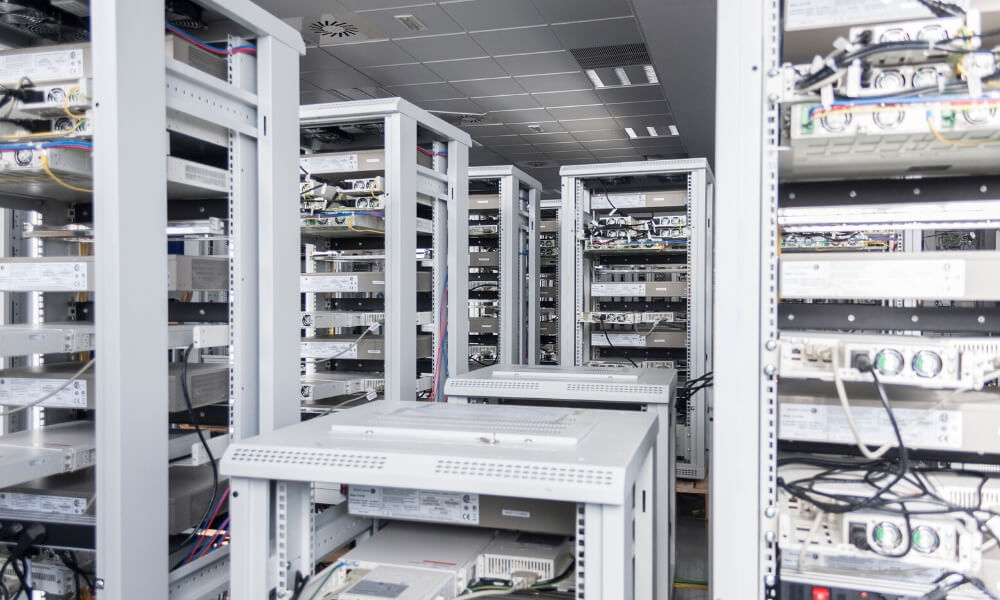In this post, we will learn about using a nonlinear correlation estimation function in R. We will also look at a few examples.

## Background

Correlation estimations are commonly used in various data mining applications. In my experience, nonlinear correlations are quite common in various processes. Due to this, nonlinear models, such as SVM, are employed for regression, classification, etc. However, there are not many approaches to estimate nonlinear correlations between two variables.

Typically linear correlations are estimated. However, the data may have a nonlinear correlation but little to no linear correlation. In such cases, nonlinearly correlated variables are sometimes overlooked during data exploration or variable selection in high-dimensional data.

We have developed a new nonlinear correlation estimator:

`nlcor`

This estimator comes useful in data exploration and also variable selection for nonlinear predictive models, such as SVM.

## Installing

To install

`nlcor`

1. Install the devtools package. You can do this from CRAN. You can do it directly in R console by typing,

3. Install

`nlcor`

from its GitHub repository by typing this in R console.

## Nonlinear Correlation Estimator: Nlcor

In this package, we provide an implementation of a nonlinear correlation estimation method using an adaptive local linear correlation computation in

`nlcor`

The function

`nlcor`

returns the nonlinear correlation estimate, the corresponding adjusted p-value, and an optional plot visualizing the nonlinear relationships.

The correlation estimate will be between 0 and 1. The higher the value the more is the nonlinear correlation. Unlike linear correlations, a negative value is not valid here. Due to multiple local correlation computations, the net p-value of the correlation estimate is adjusted (to avoid false positives). The plot visualizes the local linear correlations.

In the following, we will show its usage with a few examples. In the given examples, the linear correlations between

`x`

and

`nlcor`

is small, however, there is a visible nonlinear correlation between them. This package contains the data for these examples and can be used for testing the package.

`nlcor`

package has few sample

`x`

and

`y`

vectors that are demonstrated in the following examples.

First, we will load the package.

## Example 1. A data with cyclic nonlinear correlation.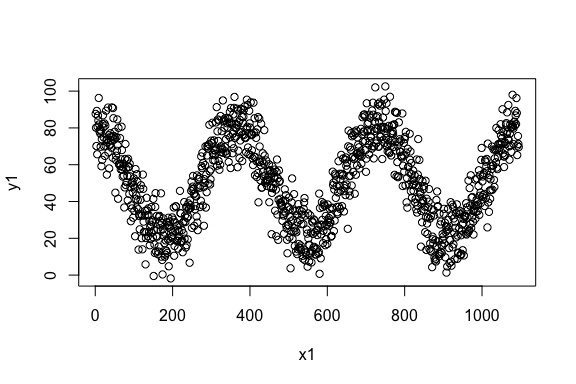The linear correlation of the data is,

As expected, the correlation is close to zero. We estimate the nonlinear correlation usingThe plot shows the piecewise linear correlations present in the data.

## Example 2. A data with non-uniform piecewise linear correlations.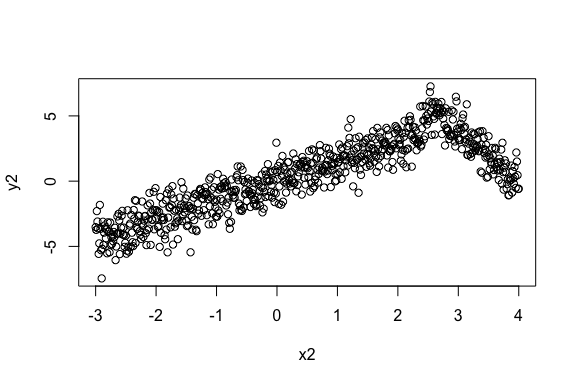The linear correlation of the data is,

The linear correlation is quite high in this data. However, there is significant and higher nonlinear correlation present in the data. This data emulates the scenario where the correlation changes its direction after a point. Sometimes that change point is in the middle causing the linear correlation to be close to zero. Here we show an example when the change point is off-center to show that the implementation works in non-uniform cases.

We estimate the nonlinear correlation usingIt is visible from the plot that

could estimate the piecewise correlations in a non-uniform scenario. Also, the nonlinear correlation comes out to be higher than the linear correlation.

## Example 3. A data with higher and multiple frequency variations.The linear correlation of the data is,

The linear correlation is expectedly small, albeit not close to zero due to some linearity.

Here we show we can refine the granularity of the correlation computation.

Under default settings, the output of

will beAs can be seen in the figure,

could identify the granular piecewise correlations. In this data, the p-value still remains extremely small—the correlation is statistically significant.

## Summary

This package provides an implementation of an efficient heuristic to compute the nonlinear correlations between numeric vectors. The heuristic works by adaptively identifying multiple local regions of linear correlations to estimate the overall nonlinear correlation. Its usages are demonstrated here with few examples.

## Artificial Intelligence Platform Achieves Unprecedented Autonomous Chemistry Control for Tissue Mill

The goal of this project was to autonomously control part of a tissue mill’s continuous manufacturing process using artificial intelligence and predictive analytics to reduce raw material consumption while maintaining the product quality with specification limit.

## LSTM Autoencoder for Extreme Rare Event Classification in Keras

Here we will learn the details of data preparation for LSTM models, and build an LSTM Autoencoder for rare-event classification. This post is a continuation of my previous post-Extreme Rare Event Classification using Autoencoders. In the previous post, we talked about the challenges in an extremely rare event data with less than 1% positively labeled data.

## Extreme Rare Event Classification using Autoencoders in Keras

In a rare-event problem, we have an unbalanced dataset. Meaning, we have fewer positively labeled samples than negative. In a typical rare-event problem, the positively labeled data are around 5–10% of the total. In an extremely rare event problem, we have less than 1% positively labeled data.

## Sequence Embedding for Clustering and Classification

Sequence Embedding for Clustering and Classification#### Case Study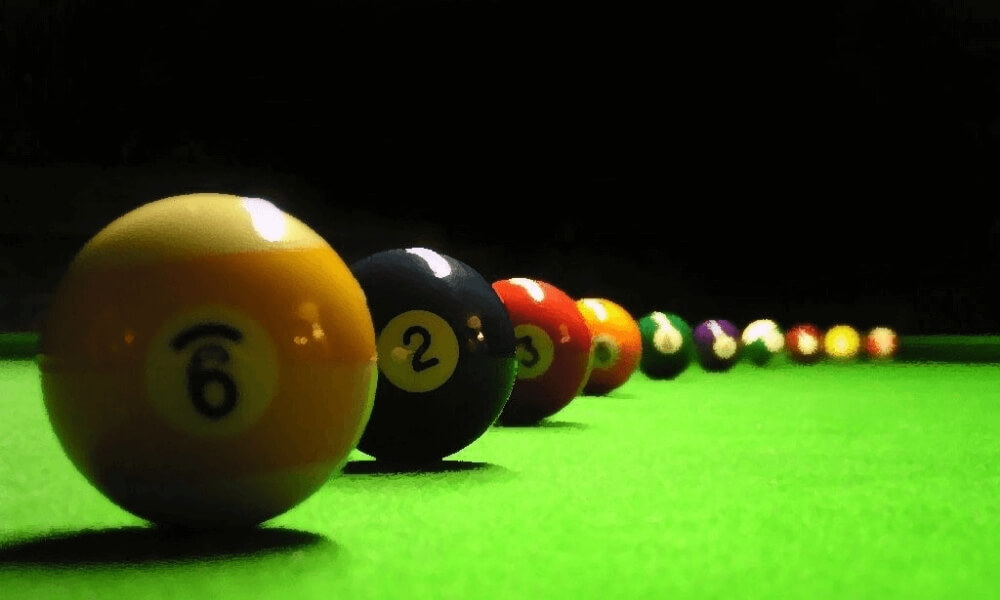Here we will learn an approach to get vector embeddings for string sequences. These embeddings can be used for Clustering and Classification.

Sequence modeling has been a challenge. This is because of the inherent un-structuredness of sequence data. Just like texts in Natural Language Processing (NLP), sequences are arbitrary strings. For a computer, these strings have no meaning. As a result, building a data mining model is difficult.

For texts, we have come up with embeddings, such as word2vec, that convert a word into an n-dimensional vector. Essentially, bringing it in a Euclidean space. In this post, we will learn to do the same for sequences.

Here we will go over an approach to create embeddings for sequences that bring a sequence in a Euclidean space. With these embeddings, we can perform conventional Machine Learning and Deep Learning, e.g. K-means, PCA, and Multi-Layer Perceptron on sequence datasets. We provide and work on two datasets — protein sequences and weblogs.

Sequence datasets are commonly found around us. For example, clickstreams, music listening history, and weblogs in tech industries. In BioInformatics, we have large databases of protein sequences. A protein sequence is made of some combination of 20 amino acids. A typical protein sequence is shown where each letter corresponds to an amino acid.

A protein sequence does not necessarily contain all the 20 amino acids but some subset of it.

For clarity, we will define some keywords used in this post.

• alphabet: the discrete elements that make up a sequence. E.g. an amino acid.
• alphabet-set: a set of all alphabets that will make sequences in a corpus. E.g. all protein sequences in a corpus are made of a set of 20 amino acids.
• sequence: an ordered series of discrete alphabets. A sequence in a corpus contains a subset of alphabet-set.

The sequence corpus typically contains thousands to millions of sequences. Clustering and Classification are often required given we have labeled or unlabeled data. However, doing this is not straightforward due to the un-structuredness of sequences — arbitrary strings of arbitrary length.

To overcome this, sequence embeddings can be used. Here we will use an SGT embedding that embeds the long- and short- term patterns in a sequence into a finite-dimensional vector. The advantage of SGT embedding is that we can easily tune the amount of long- / short- term patterns without increasing the computation.

The source code and data in the following is . Before moving forward, we will need to install Sgt package:

sgt

\$ pip install sgt

## Clustering

### Protein Sequence Clustering

The data used here is taken from . This is a public database for proteins. The data contains the protein sequences and their function. In this section, we will cluster the protein sequences, and in the next we will use their functions as labels for building a classifier.

We first read the sequence data, and convert it into a list of lists. As shown below, each sequence is a list of alphabets.

```>>> protein_data = pd.DataFrame.from_csv('../data/protein_classification.csv')
>>> X = protein_data['Sequence']
>>> def split(word):
>>>    return [char for char in word]>>> sequences = [split(x) for x in X]
>>> print(sequences)
['M', 'E', 'I', 'E', 'K', 'T', 'N', 'R', 'M', 'N', 'A', 'L', 'F', 'E', 'F', 'Y', 'A', 'A', 'L', 'L', 'T', 'D', 'K', 'Q', 'M', 'N', 'Y', 'I', 'E', 'L', 'Y', 'Y', 'A', 'D', 'D', 'Y', 'S', 'L', 'A', 'E', 'I', 'A', 'E', 'E', 'F', 'G', 'V', 'S', 'R', 'Q', 'A', 'V', 'Y', 'D', 'N', 'I', 'K', 'R', 'T', 'E', 'K', 'I', 'L', 'E', 'D', 'Y', 'E', 'M', 'K', 'L', 'H', 'M', 'Y', 'S', 'D', 'Y', 'I', 'V', 'R', 'S', 'Q', 'I', 'F', 'D', 'Q', 'I', 'L', 'E', 'R', 'Y', 'P', 'K', 'D', 'D', 'F', 'L', 'Q', 'E', 'Q', 'I', 'E', 'I', 'L', 'T', 'S', 'I', 'D', 'N', 'R', 'E']```

Next, we generate the sequence embeddings.

```>>> from sgt import Sgt
>>> sgt = Sgt(kappa = 10, lengthsensitive = False)
>>> embedding = sgt.fit_transform(corpus=sequences)```

The embedding is in 400-dimensional space. Let’s first do PCA on it and reduce the dimension to two. This will also help visualize the clusters.

```>>> pca = PCA(n_components=2)
>>> pca.fit(embedding)
>>> X = pca.transform(embedding)>>> print(np.sum(pca.explained_variance_ratio_))
0.6019403543806409```

The top two PCs are explaining about 60% of the variance. We will cluster them into 3 clusters.

```>>> kmeans = KMeans(n_clusters=3, max_iter =300)
>>> kmeans.fit(df)>>> labels = kmeans.predict(df)
>>> centroids = kmeans.cluster_centers_>>> fig = plt.figure(figsize=(5, 5))
>>> colmap = {1: 'r', 2: 'g', 3: 'b'}
>>> colors = list(map(lambda x: colmap[x+1], labels))
>>> plt.scatter(df['x1'], df['x2'], color=colors, alpha=0.5, edgecolor=colors)```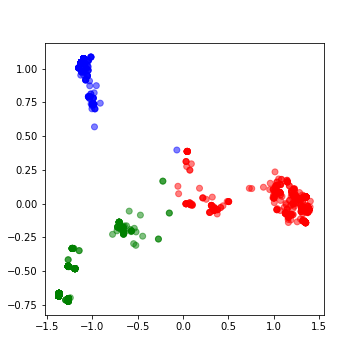Moving on to building classifiers.

## Classification

### Protein Sequence Classification

We will start by building a classifier on the same protein dataset we used earlier. The proteins in the dataset have two functions. Therefore, we will build a binary classifier.

We will first convert the:

`function [CC]`

a column in the data into labels that can be ingested in an MLP model built-in

`keras`
```>>> y = protein_data['Function [CC]']
>>> encoder = LabelEncoder()
>>> encoder.fit(y)
>>> encoded_y = encoder.transform(y)```

In the following, we build the MLP classifier and run 10-fold cross-validation.

```>>> kfold = 10
>>> X = pd.DataFrame(embedding)
>>> y = encoded_y>>> random_state = 1>>> test_F1 = np.zeros(kfold)
>>> skf = KFold(n_splits = kfold, shuffle = True, random_state = random_state)
>>> k = 0
>>> epochs = 50
>>> batch_size = 128>>> for train_index, test_index in skf.split(X, y):
>>>     X_train, X_test = X.iloc[train_index], X.iloc[test_index]
>>>     y_train, y_test = y[train_index], y[test_index]
>>>     X_train = X_train.as_matrix(columns = None)
>>>     X_test = X_test.as_matrix(columns = None)
>>>
>>>     model = Sequential()
>>>     model.add(Dense(64, input_shape = (X_train.shape,), init = 'uniform'))
>>>
>>>     model.fit(X_train, y_train ,batch_size=batch_size, epochs=epochs, verbose=0)
>>>
>>>     y_pred = model.predict_proba(X_test).round().astype(int)
>>>     y_train_pred = model.predict_proba(X_train).round().astype(int)>>>     test_F1[k] = sklearn.metrics.f1_score(y_test, y_pred)
>>>     k+=1

>>> print ('Average test f1-score', np.mean(test_F1))
Average test f1-score 1.0```

This data turns out to be too good for the classifier. We have another dataset that is more challenging. Let’s go over it.

### Network Log Data Classification

This data sample is taken from . This is a network intrusion data containing audit logs and any attack as a positive label. Since network intrusion is a rare event, the data is unbalanced. Additionally, we have a small dataset of only 111 records. Here we will build a sequence classification model to predict a network intrusion.

Each sequence contains in the data is a series of activity, for example,

`{login, password, …}`

The alphabets in the input data sequences are already encoded into integers. The original sequences data file is present .

Similar to before, we will first prepare the data for a classifier.

```>>> darpa_data = pd.DataFrame.from_csv('../data/darpa_data.csv')
>>> X = darpa_data['seq']
>>> sequences = [x.split('~') for x in X]>>> y = darpa_data['class']
>>> encoder = LabelEncoder()
>>> encoder.fit(y)
>>> y = encoder.transform(y)```

In this data, the sequence embeddings should be length-sensitive. The lengths are important here because sequences with similar patterns but different lengths can have different labels. Consider a simple example of two sessions:

`{login, pswd, login, pswd,…}{login, pswd,…(repeated several times)…, login, pswd}`

While the first session can be a regular user mistyping the password once, the other session is possibly an attack to guess the password. Thus, the sequence lengths are as important as the patterns.

```>>> sgt_darpa = Sgt(kappa = 5, lengthsensitive = True)
>>> embedding = sgt_darpa.fit_transform(corpus=sequences)```

The embedding we find here is sparse. Therefore, we will perform dimension reduction using PCA before we train a classifier.

```>>> from sklearn.decomposition import PCA
>>> pca = PCA(n_components=35)
>>> pca.fit(embedding)
>>> X = pca.transform(embedding)
>>> print(np.sum(pca.explained_variance_ratio_))
0.9862350164327149```

The selected top-35 PCAs are explaining more than 98% of the variance. We will now go ahead and build a Multi-Layer Perceptron using keras. Since the data size is small and, also, the number of positive labeled points, we will perform a 3-fold validation.

```>>> kfold = 3
>>> random_state = 11>>> test_F1 = np.zeros(kfold)
>>> time_k = np.zeros(kfold)
>>> skf = StratifiedKFold(n_splits=kfold, shuffle=True, random_state=random_state)
>>> k = 0
>>> epochs = 300
>>> batch_size = 15>>> class_weight = {0 : 0.12, 1: 0.88,}  # The weights can be changed and made inversely proportional to the class size to improve the accuracy.>>> for train_index, test_index in skf.split(X, y):
>>>     X_train, X_test = X[train_index], X[test_index]
>>>     y_train, y_test = y[train_index], y[test_index]
>>>
>>>     model = Sequential()
>>>     model.summary()
>>>
>>>     start_time = time.time()
>>>     model.fit(X_train, y_train ,batch_size=batch_size, epochs=epochs, verbose=1, class_weight=class_weight)
>>>     end_time = time.time()
>>>     time_k[k] = end_time-start_time>>>     y_pred = model.predict_proba(X_test).round().astype(int)
>>>     y_train_pred = model.predict_proba(X_train).round().astype(int)
>>>     test_F1[k] = sklearn.metrics.f1_score(y_test, y_pred)
>>>     k += 1
>>> print ('Average Test f1-score', np.mean(test_F1))
Average Test f1-score 0.5236467236467236>>> print ('Average Run time', np.mean(time_k))
Average Run time 9.076935768127441```

This was a difficult data to classify. To have a loose benchmark, let’s build a fancier LSTM classifier on the same data.

```>>> X = darpa_data['seq']
>>> encoded_X = np.ndarray(shape=(len(X),), dtype=list)
>>> for i in range(0,len(X)):
>>>     encoded_X[i]=X.iloc[i].split("~")
>>> max_seq_length = np.max(darpa_data['seqlen'])
>>> encoded_X = sequence.pad_sequences(encoded_X, maxlen=max_seq_length)>>> kfold = 3
>>> random_state = 11>>> test_F1 = np.zeros(kfold)
>>> time_k = np.zeros(kfold)>>> epochs = 50
>>> batch_size = 15
>>> skf = StratifiedKFold(n_splits=kfold, shuffle=True, random_state=random_state)
>>> k = 0>>> for train_index, test_index in skf.split(encoded_X, y):
>>>     X_train, X_test = encoded_X[train_index], encoded_X[test_index]
>>>     y_train, y_test = y[train_index], y[test_index]
>>>
>>>     embedding_vecor_length = 32
>>>     top_words=50
>>>     model = Sequential()
>>>     model.compile(loss='binary_crossentropy', optimizer='adam', metrics=['accuracy'])>>>     start_time = time.time()
>>>     model.fit(X_train, y_train, epochs=epochs, batch_size=batch_size, verbose=1)
>>>     end_time=time.time()
>>>     time_k[k]=end_time-start_time>>>     y_pred = model.predict_proba(X_test).round().astype(int)
>>>     y_train_pred=model.predict_proba(X_train).round().astype(int)
>>>     test_F1[k]=sklearn.metrics.f1_score(y_test, y_pred)
>>>     k+=1>>> print ('Average Test f1-score', np.mean(test_F1))
Average Test f1-score 0.0>>> print ('Average Run time', np.mean(time_k))
Average Run time 425.68603706359863```

We find that the LSTM classifier gives an F1 score of 0. This may be improved by changing the model. However, we find that the SGT embedding could work with small and unbalanced data without the need of a complicated classifier. Besides, on the runtime, SGT embedded network was significantly faster. It took on average 9.1 secs while the LSTM model took 425.6 secs.

### Concluding Remarks

• We learned about using a sequence embedding for sequence clustering and classification.
• This embedding is an implementation of this . Not covered in this post, but refer to this paper to see the accuracy comparison of SGT embedding over others.
• Due to SGT embedding’s ability to capture long- and short- term patterns, it works better than most other sequence modeling approaches.
• It is recommended that you play with the tuning parameter, kappa, to see its effect on accuracy.

## Artificial Intelligence Platform Achieves Unprecedented Autonomous Chemistry Control for Tissue Mill

The goal of this project was to autonomously control part of a tissue mill’s continuous manufacturing process using artificial intelligence and predictive analytics to reduce raw material consumption while maintaining the product quality with specification limit.

## LSTM Autoencoder for Extreme Rare Event Classification in Keras

Here we will learn the details of data preparation for LSTM models, and build an LSTM Autoencoder for rare-event classification. This post is a continuation of my previous post-Extreme Rare Event Classification using Autoencoders. In the previous post, we talked about the challenges in an extremely rare event data with less than 1% positively labeled data.

## Extreme Rare Event Classification using Autoencoders in Keras

In a rare-event problem, we have an unbalanced dataset. Meaning, we have fewer positively labeled samples than negative. In a typical rare-event problem, the positively labeled data are around 5–10% of the total. In an extremely rare event problem, we have less than 1% positively labeled data.